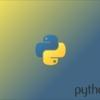## Welcome to the Treehouse Community

The Treehouse Community is a meeting place for developers, designers, and programmers of all backgrounds and skill levels to get support. Collaborate here on code errors or bugs that you need feedback on, or asking for an extra set of eyes on your latest project. Join thousands of Treehouse students and alumni in the community today. (Note: Only Treehouse students can comment or ask questions, but non-students are welcome to browse our conversations.)

### Looking to learn something new?

Treehouse offers a seven day free trial for new students. Get access to thousands of hours of content and a supportive community. Start your free trial today.# messy code

since we cant use a string for the key, is this the only way to do the challenge?:

time_machine.py
```import datetime

starter = datetime.datetime(2015, 10, 21, 16, 29)

# Remember, you can't set "years" on a timedelta!
# Consider a year to be 365 days.

## Example
# time_machine(5, "minutes") => datetime(2015, 10, 21, 16, 34)

def time_machine(integer, string):
if string == 'years':
return starter + datetime.timedelta(days = integer * 365)
elif string == 'days':
return starter + datetime.timedelta(days = integer)
elif string == 'weeks':
return starter + datetime.timedelta(weeks = integer)
elif string == 'seconds':
return starter + datetime.timedelta(seconds = integer)
elif string == 'microseconds':
return starter + datetime.timedelta(microseconds = integer)
elif string == 'milliseconds':
return starter + datetime.timedelta(milliseconds = integer)
elif string == 'minutes':
return starter + datetime.timedelta(minutes = integer)
elif string == 'hours':
return starter + datetime.timedelta(hours = integer)
```

Hi Kevin,

Your code could be about half as short since the challenge states that only "minutes", "hours", "days", or "years" will be passed in.

Beyond that, you could create a dictionary out of the `string`, and `integer` and then unpack the dictionary when you pass it into timedelta. This sets up the proper keyword argument while avoiding the problem of not being able to use a string as the key.

Since "years" is not a valid keyword argument, you first have to check for that and do some adjusting.

```def time_machine(integer, string):
if string == "years":
# if years were passed in then adjust the variables to represent days instead
string = "days"
integer *= 365
return starter + datetime.timedelta(**{string : integer}) # create a dictionary with string and integer and then unpack it
```